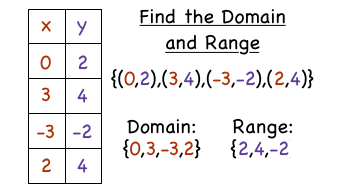# Domain and Range of a Function

## Key Questions

See below

#### Explanation:

I will assume $\left\{f \left(x\right) , x\right\} \in \mathbb{R}$

Then, the domain of $f \left(x\right)$ is the set of all real values of $x$ for which $f \left(x\right)$ is defined. We can think of this as the valid inputs. Let's now call this set $D$

Then the range of $f \left(x\right)$ is the set of values of $f \left(x\right)$ over $D$. We can think of this as the valid outputs.

To determine the domain and range of a function, first determine the set of values for which the function is defined and then determine the set of values which result from these.

E.g. $f \left(x\right) = \sqrt{x}$

$f \left(x\right)$ is defined $\forall x \ge 0 : f \left(x\right) \in \mathbb{R}$

Hence, the domain of $f \left(x\right)$ is $\left[0 , + \infty\right)$

Also, $f \left(0\right) = 0$ and $f \left(x\right)$ has no finite upper bound.

Hence, the range of $f \left(x\right)$ is also $\left[0 , + \infty\right)$

We can deduce these results from the graph of $\sqrt{x}$ below.

graph{sqrtx [-4.18, 21.13, -6.51, 6.15]}

• None of the two, actually. However, the domain is related to the independent variable, as it is the set of all the "permitted" values for the independent variable to assume. In particular, given a function, you must be sure that:

1. If there is a fraction, the denominator can't be zero;
2. If there is an even root, its argument must be positive or zero;
3. If there is a logarithm, its argument must be strictly positive.

These requests can of course be combined, as for example in $\setminus \sqrt{\setminus \log \left(\setminus \frac{x}{x + 1}\right)}$.

Once you find out which values of $x$ are permitted for the expression to have sense, you take the set of all those values, and obtain the domain of the function.

• There are so many different kinds of functions, but domain and range are important parts of your study of functions.

Let me give you some examples of polynomial functions:
y = 3x + 1, y = ${x}^{2} + 3 x + 2$, and y = ${x}^{3}$. Do you notice that each one of those functions has powers of x that are Whole numbers? Stick with those, and you will have a polynomial.

All polynomials have a domain of "All Real Numbers". In interval notation, we write: $\left(- \setminus \infty , \setminus \infty\right)$. On the horizontal number line, that covers all numbers from left to right (your x-axis).

Polynomials with ODD degree (highest power of x) stretch their way from low to high through all real numbers in the vertical direction. This means that their Range is "All Real Numbers" again: $\left(- \setminus \infty , \setminus \infty\right)$. Once these functions get going in those directions, you will never see the end of them! We call this their "End behavior".

Polynomials with EVEN degree must have either a maximum or minimum value. If the graph has a minimum value, then its y-values (Range) stretch from that number, up to $\setminus \infty$. We write that Range as $\left[\min , \setminus \infty\right)$. Look at the graph shown below, it has a minimum (vertex) at (2,-4). Its Range would be $\left[- 4 , \setminus \infty\right)$.Notice that whenever we use the $\setminus \infty$ symbols, we use a round ( or ). That means that we can not include a numeric value for the infinities. When we use the square [ or ], it refers to an actual value that is included in the function.

Your study of domain and range has just begun, and will include a wide variety of functions besides polynomials. When you discover a new function that behaves differently, carefully analyze its input and output values. You are on the way to great things!

• First, let's define a function:
A function is a relationship between the $x$ and $y$ values, where each $x$-value or input has only one $y$-value or output .

Domain: all x-values or inputs that have an output of real $y$-values.

Range: the y-values or outputs of a functionFor example,